# Graphing Calculator Mathlab PRO v4.9.130 Apk

If you're looking for a graphing calculator app that works smoothly and seamlessly, you've found it! Graphing Calculator by Ma...

If you're looking for a graphing calculator app that works smoothly and seamlessly, you've found it! Graphing Calculator by Mathlab is a scientific graphing calculator integrated with algebra and is an indispensable mathematical tool for students in elementary school to those in college or graduate school, or just anyone who needs more than what a basic calculator offers. It is designed to replace bulky and costly handheld graphing calculators and works on virtually any Android phone or tablet.
Furthermore, Graphing Calculator by Mathlab displays calculations as it performs them on the high-quality display of the Android device, making it easier for the user to understand the calculations and see them clearly. This app has two great strengths. First, it acts as a fine scientific calculator, but, more than that, it displays the intermediate steps of the calculations as you type. It allows the students to both watch and learn how the calculations are made and how to find the final answer. Second, the graphing ability is absolutely stunning! Not only does the calculator beautifully display the graphs, but it automatically generates the x- and y- values and displays them as well.
Video: https://youtu.be/6BR8Lv1U9kA
Help site with instructions and examples: http://help.mathlab.us
If you have a question, send email to calc@mathlab.us
PRO FEATURES
* 3D graphs
* Full screen
* 9 workspaces
* Save constants, functions and expressions in the library
* Internet is not required
SCIENTIFIC CALCULATOR
* Arithmetic expressions +, -, *, /, ÷
* Square root, cube and higher roots (hold ‘√’ key)
* Exponent, logarithms (ln, log)
* Trigonometric functions sin π/2, cos 30°, ...
* Hyperbolic functions sinh, cosh, tanh, ... (hold "e" key to switch)
* Inverse functions (hold direct function key)
* Complex numbers, all functions support complex arguments
* Derivatives sin x' = cos x, ... (hold x^n key)
* Scientific notation (enable in menu)
* Percent mode
GRAPHING CALCULATOR
* Multiple functions graphing
* Implicit functions up to 2nd degree (ellipse 2x^2+3y^2=1, etc.)
* Polar graphs (r=cos2θ)
* Parametric functions, enter each on new line (x=cos t, y=sin t)
* Function roots and critical points on a graph. Select the checkbox on the left from the function to show their coordinates on the graph, click the graph-button on the upper menu to display their coordinates as a list.
* Graph intersections (x^2=x+1)
* Tracing function values and slopes
* Scrollable and resizable graphs
* Pinch to zoom
* Fullscreen graphs in landscape orientation
* Function tables
* Dave graphs as images
* Save tables as csv
FRACTION CALCULATOR
* Simple and complex fractions 1/2 + 1/3 = 5/6
* Mixed numbers, use space to enter values 3 1/2
ALGEBRA CALCULATOR
* Linear equations x+1=2 -> x=1
* Quadratic equations x^2-1=0 -> x=-1,1
* Approximate roots of higher polynomials
* Systems of linear equations, write one equation per line, x1+x2=1, x1-x2=2
* Polynomial long division
* Polynomial expansion, factoring
MATRIX CALCULATOR
* Matrix and vector operations
* Dot product (hold *), cross product
* Determinant, inverse, norm, transpose, trace
LIBRARY
* User defined constants and functions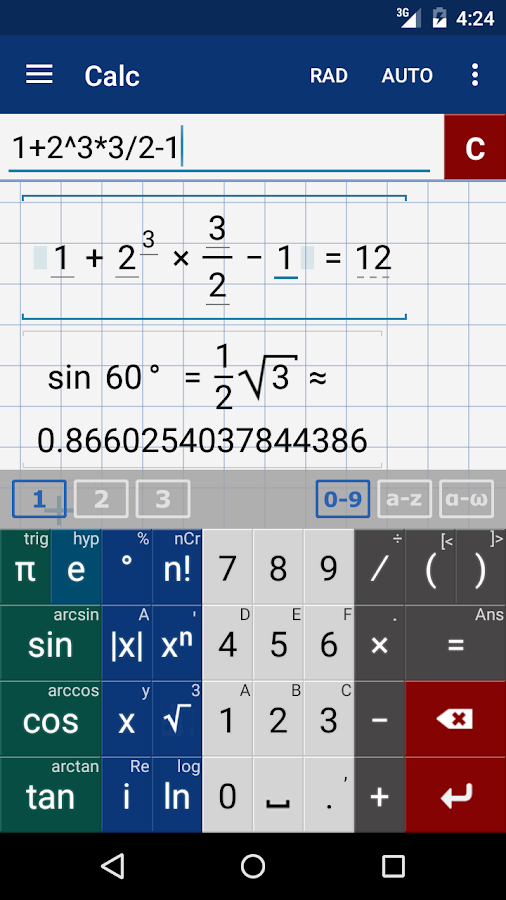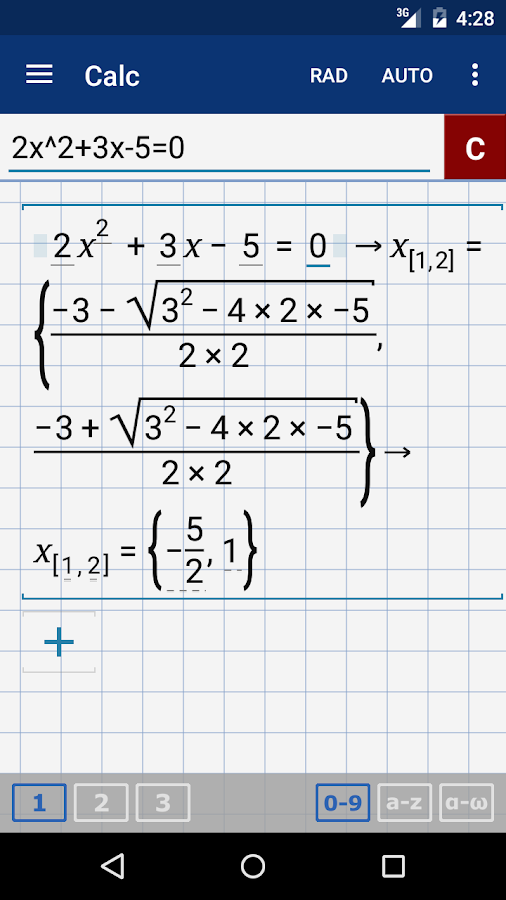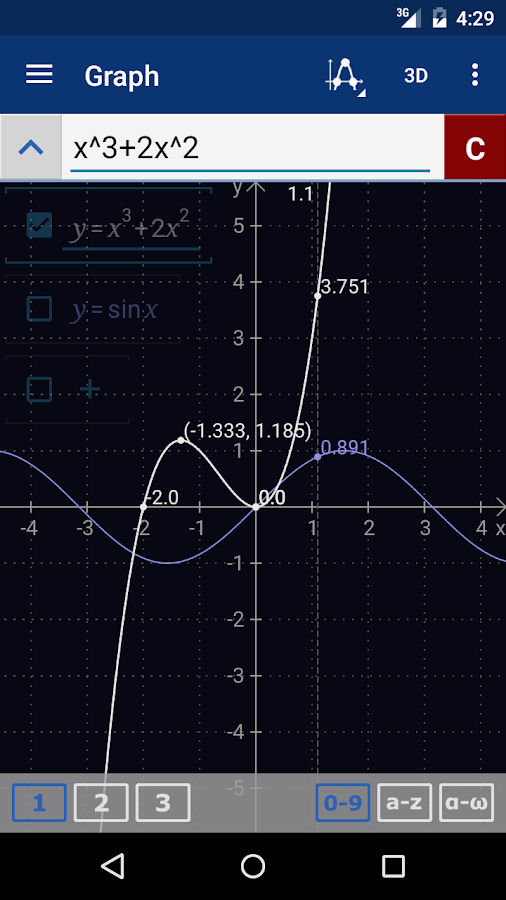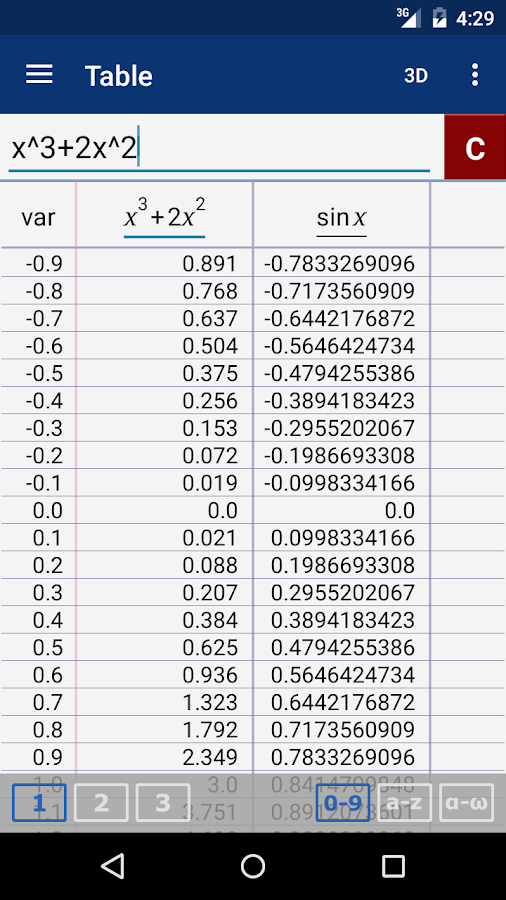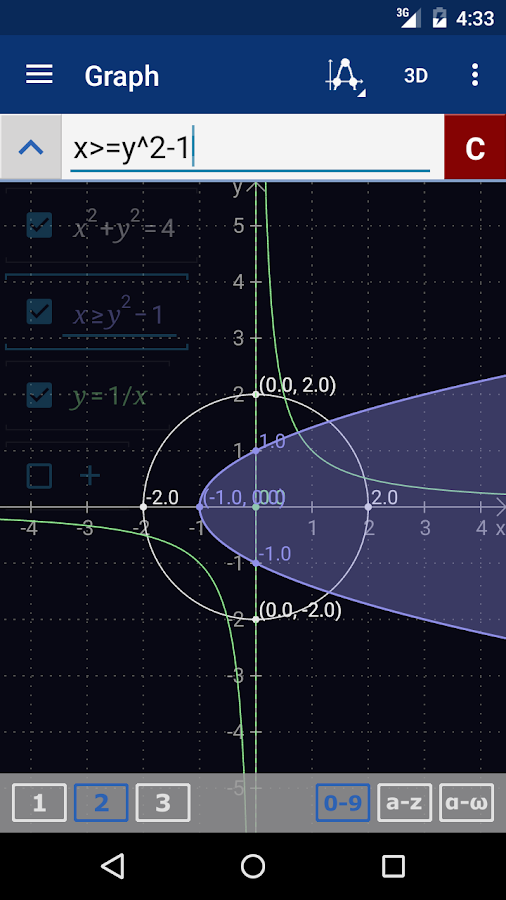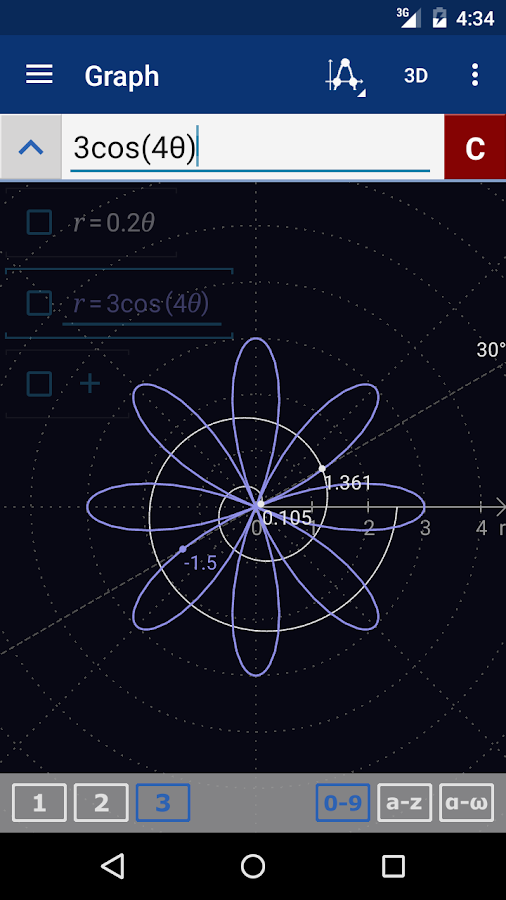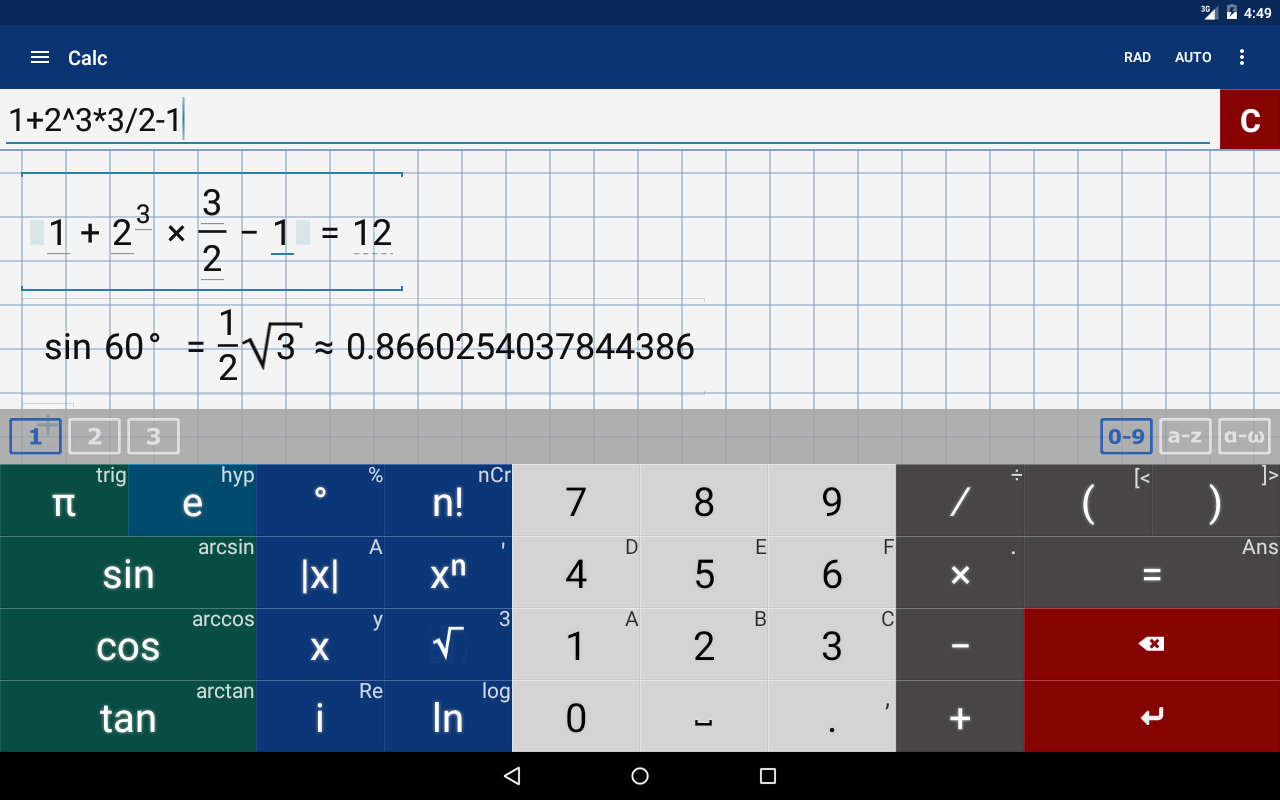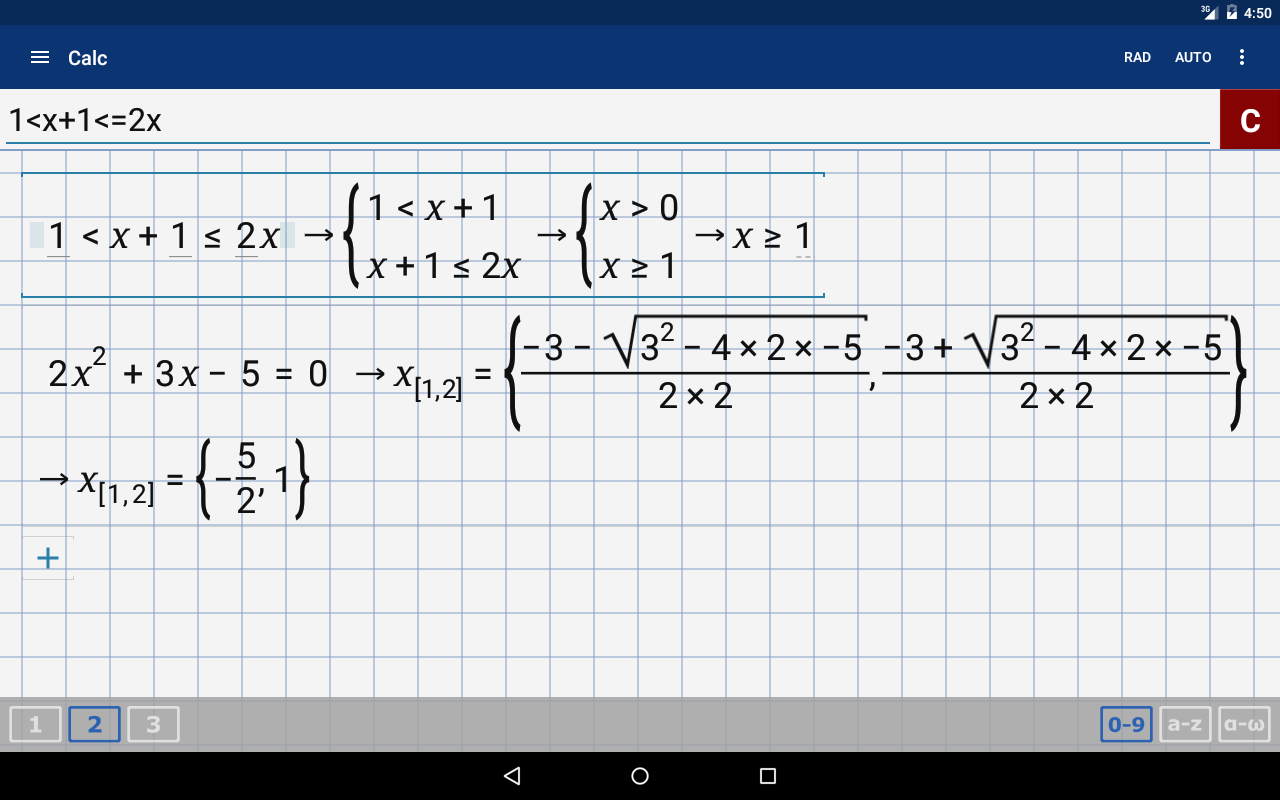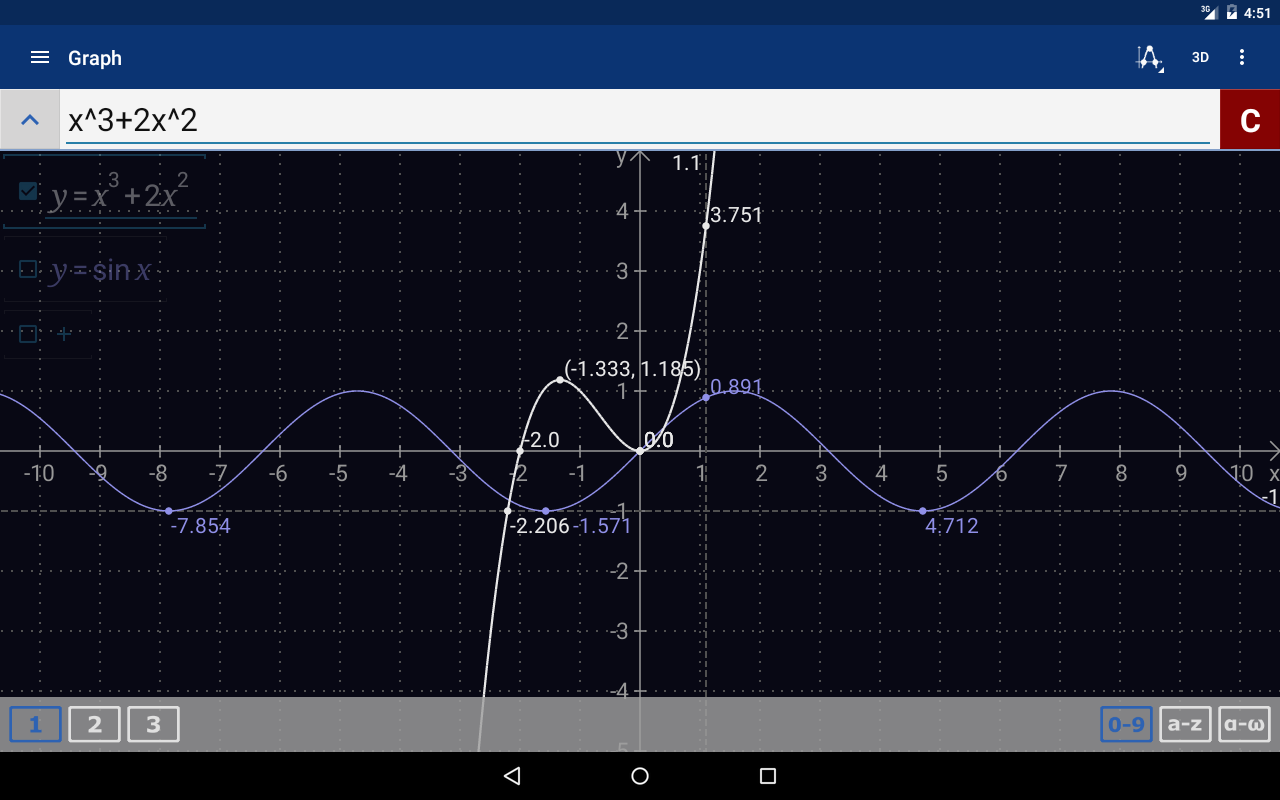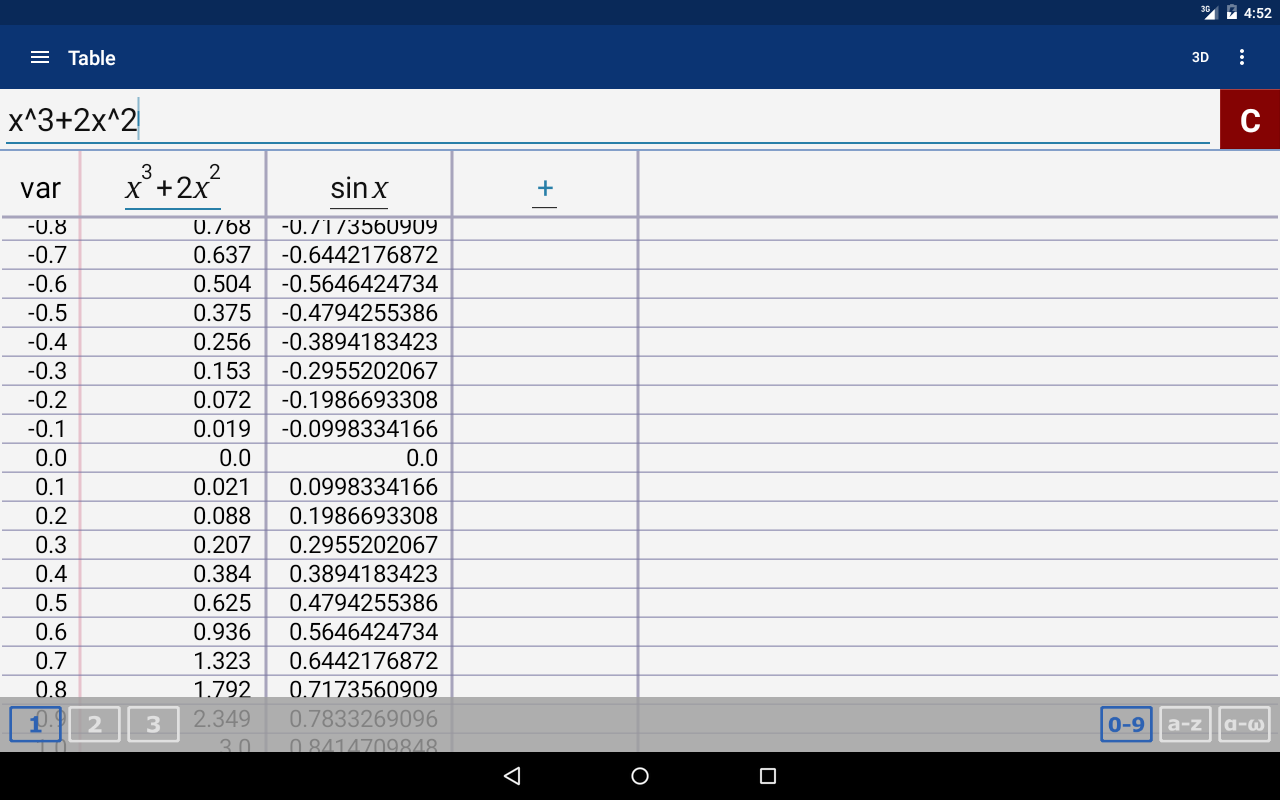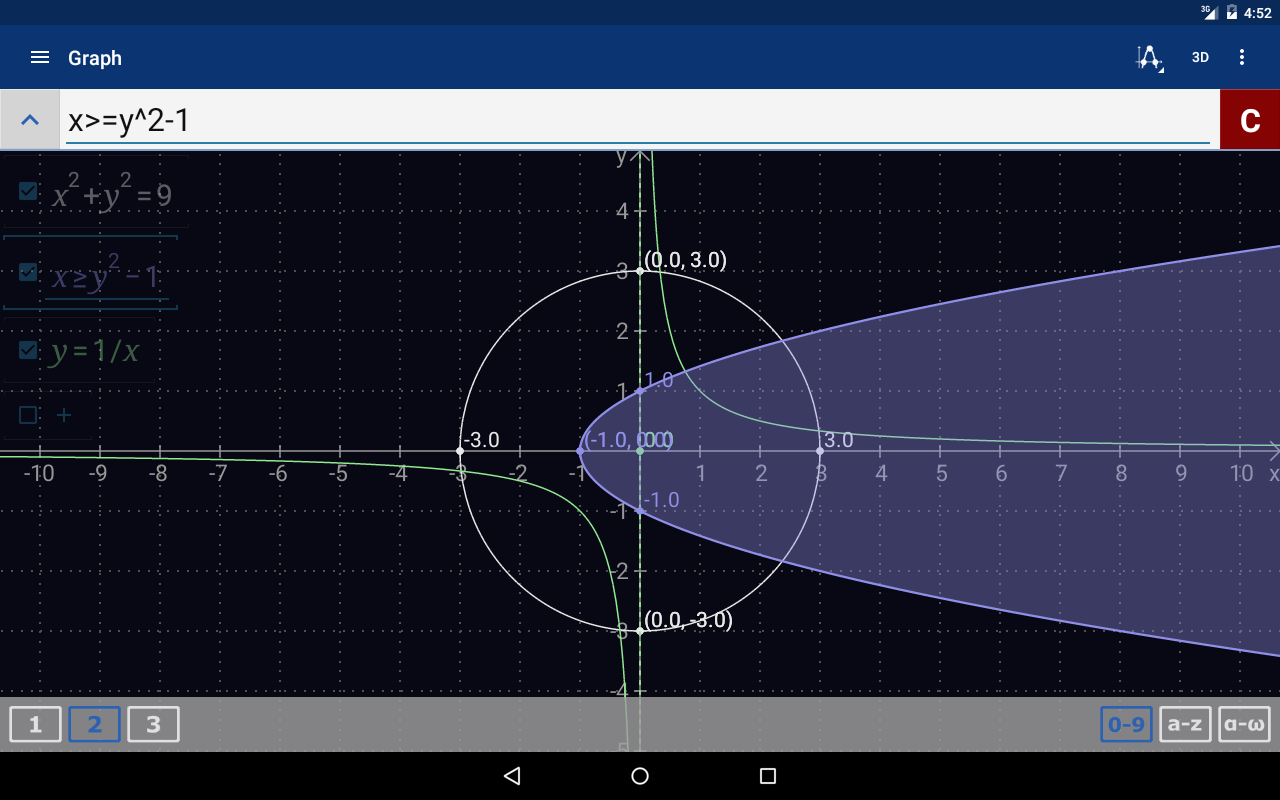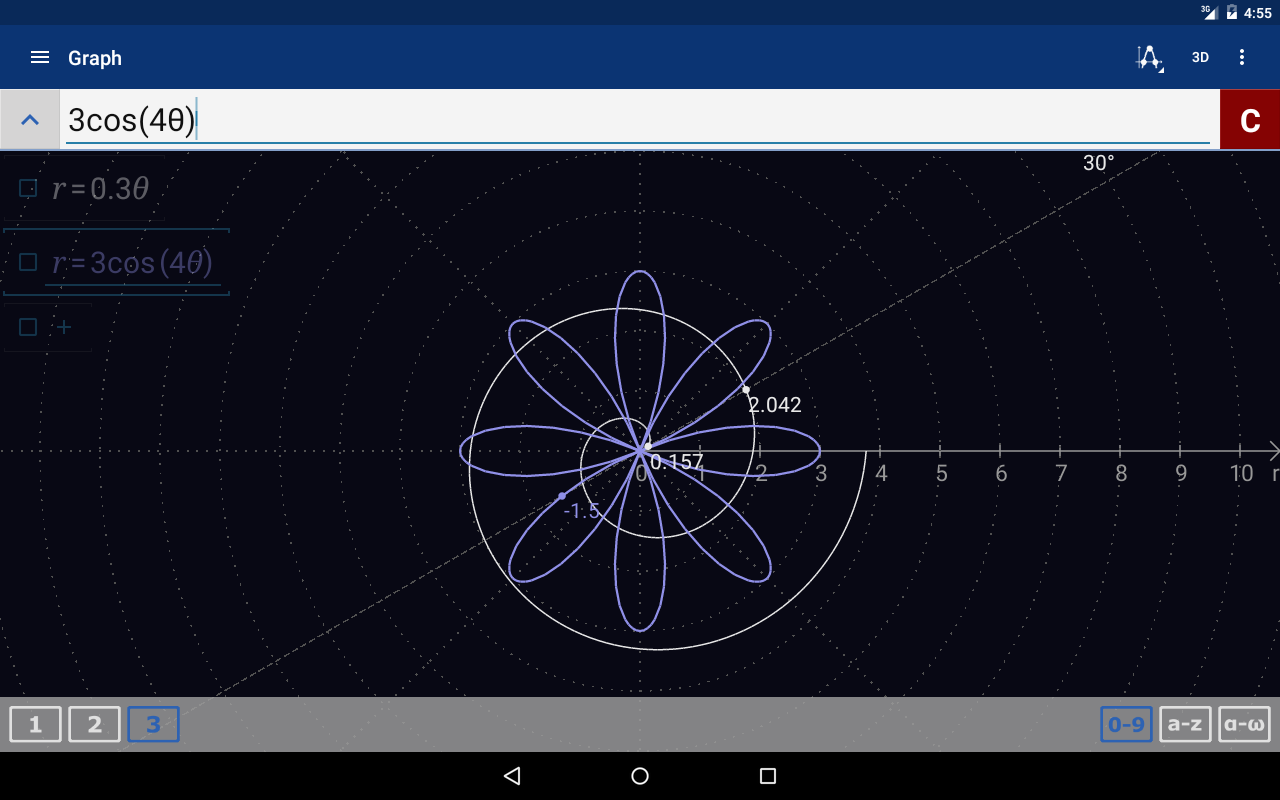# Exact values for trigonometric functions sin(60°) = 1/2*sqrt(3) Polar form for complex numbers 1∠30°, hold i to enter ∠ Visual editing (beta), enable in Settings > Calculator Fractional part - frac(1.5) = 0.5 Gamma function and related - gamma(x)/Γ(x), lngamma(x)/lnΓ(x), digamma(x)/ψ(x)

Requires Android-Varies with device